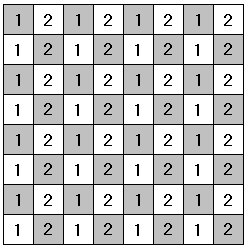# Vertical and Horizontal Dominoes on a Chessboard

Here's a nice problem with domino covering of a chessboard [Kozlova, #110]:

An 8×8 chessboard is tiled with 2×1 dominoes. Prove that the number of horizontal pieces is even and so is the number of vertical pieces.

Solution

### References

1. E. G. Kozlova, Tales and Hints: Problems for a Math Circle, MCCME, 2004 (Second edition), in RussianCopyright © 1996-2018 Alexander Bogomolny

An 8×8 chessboard is tiled with 2×1 dominoes. Prove that the number of horizontal pieces is even and so is the number of vertical pieces.

### Solution 1

Consider the number of horizontal pieces in the first row. Since one domino covers one white and one black square, horizontal pieces cover an even number of squares in the first row. It follows that the number of vertical pieces with a foothold in the first row is even.

Move now to the second row. There is an even number of vertical dominoes that with the upper square in the first row and the low square in the second row. They leave an even number of squares in the second row. An even number of these is covered with horizontal pieces. It follows that there is an even number of vertical pieces that start in the second row.

Continue in this manner, we see that there is an even number of vertical dominoes that start in rows 1-7, making the total number of vertical pieces even. Since it takes 32 dominoes to cover the whole chessboard, the number of horizontal pieces is also even.

### Solution 2

Place numbers 1 and 2 into chessboard squares as shown:Observe that every horizontal pieces covers both 1 and 2, making the sum of number beneath it odd. The vertical pieces cover an even sum of numbers (either 1+1, or 2+2). The total sum of the numbers on the chessboard is 3×32 = 96 - an even number. Since, the total of the numbers covered by the vertical pieces is even, so is the total covered by the horizontal pieces. But each horizontal piece covers the total of 3, implying that their number is even.### Related materialRead more...

• Covering A Chessboard With Domino
• Dominoes on a Chessboard
• Tiling a Chessboard with Dominoes
• Straight Tromino on a Chessboard
• Golomb's inductive proof of a tromino theorem
• Tromino Puzzle: Interactive Illustration of Golomb's Theorem
• Tromino as a Rep-tile
• Tiling Rectangles with L-Trominoes
• Squares and Straight Tetrominoes
• Covering a Chessboard with a Hole with L-Trominoes
• Tromino Puzzle: Deficient Squares
• Tiling a Square with Tetrominoes Fault-Free
• Tiling a Square with T-, L-, and a Square Tetrominoes
• Tiling a Rectangle with L-tetrominoes
• Tiling a 12x12 Square with Straight Trominoes
• Bicubal Domino
•Copyright © 1996-2018 Alexander Bogomolny

 64864375